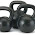## Saturday, September 10, 2011

### WOD-Max Effort 2

WOD = Max Effort Day

Work up to a max weight for each movement, using the given repetition maximum assigned.  Do so over the course of 3-5 sets for each exercise.  Once you have used the most amount of weight possible for the prescribed repetitions, you are done that exercise.  Therefore, continue on to the next movement and repeat the process of progressive resistance, ending in a max effort attempt for each one.  The max weight used in each one is what gets logged in the "comments" section below.  FYI, the weight logged needs to be the same for your right and left arm!

1) Turkish Getup - 1RM (1 repetition maximum = max weight moved for 1 repetition)
2) Front Squat - 5RM ( 5 repetition maximum = max weight moved for 5 repetition)
3) Overhead Press - 5RM ( 5 repetition maximum = max weight moved for 5 repetition)

1.8am

Tony - TGU = 24k
FS = 40k
OHP = 58#

Julie - TGU = 8k
FS = 20#
OHP = 8k

Kathy - TGU = 15#
FS = 12k
OHP = 8k

Dave - TGU = 37.5#
FS = 32k
OHP = 30#

Craig - TGU = 8k
FS = 32k (only 3 reps)
OHP = 20k

2.9am

Amy - TGU = 37.5#
FS = 24k
OHP = 20k (replaced with 1 arm jerk)

John - TGU = 58#
FS = 40k
OHP = 20k

Gabe - TGU = 16k
FS = 40k
OHP = 49#

Fritz - TGU = 58#
FS = 40k
OHP = 16k

Lina - TGU = 12k
FS = 16k
OHP = 12k

Leslie C - TGU = 10#
FS = 25#
OHP = 8k

3.10am

Karen - TGU = 15# (half getup)
FS = 25#
OHP = 15#

Mary - TGU = 15# (half getup)
FS = 30#
OHP = 15#

Marianne - TGU = 20#
FS = 16k
OHP = 20#

Jake - TGU = 35#
FS = 44k
OHP = 24k